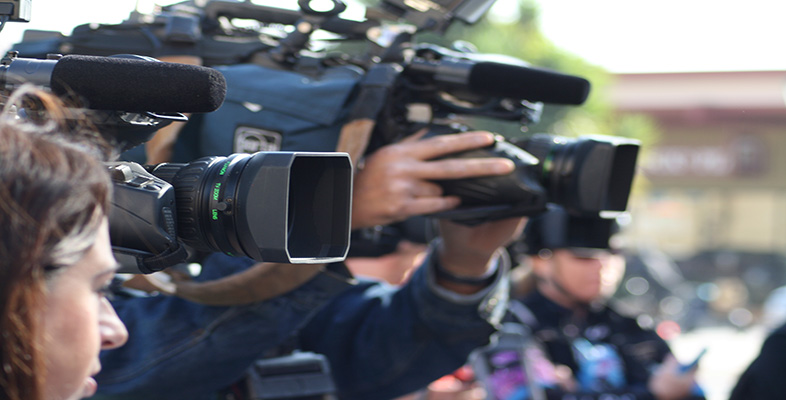IT: Information

Start this free course now. Just create an account and sign in. Enrol and complete the course for a free statement of participation or digital badge if available.

Free course

# 5.3 Signal speeds, propagation times and distance: the formula triangle

When signals travel along a wire or optical fibre, or through space, the relationship between the speed, propagation time and distance can be written in three ways, depending upon which one you want to calculate.

If you know the speed and the propagation time and want to know how far the signal will travel, you use: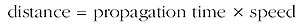You should recall that this is the calculation used for the active autofocusing discussed in Section 4.2.5.

I shall use d for the distance and t for the time, as I did earlier. For speed I shall follow the common convention of using v , which comes from 'velocity' – but you have to be careful not to get confused with v for voltage, as used in Section 4.4.

So we have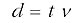Technically the value for the velocity of something includes both its speed and the direction it is moving in. For our purposes there is no need to distinguish between speed and velocity. I shall just use the word speed and the symbol v.

If you know the distance travelled and speed but want to calculate the propagation time you use: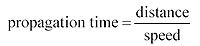which can be written: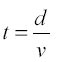Finally, if you know the distance travelled and the propagation time, and want to calculate the speed, you use: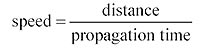or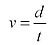These are of course quite general relationships between distance travelled, time taken and speed. We used the same relationship when doing the calculations for autofocusing in a camcorder, and it applies equally for the journey time driving along a motorway or cycling to work, assuming constant speed (or using average speed in the calculation). The relationship between these three terms is displayed on a formula triangle as shown in Figure 27. You can swap the 'time' t and 'speed' (v) in the lower corners – it doesn't matter which way round these are.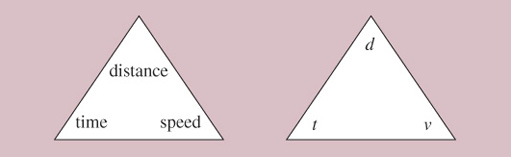Figure 27 The formula triangle

Earlier I explained how you draw the formula triangle given one of the equations, but what is useful about the triangle is that you can get back to any of the equations from the triangle.

You do this by covering the quantity you want to calculate, and looking at the position of the other two (Figure 28). So if you want to calculate the time, you cover 'time' and observe that distance appears above speed, so you calculate time from distance divided by speed. Similarly, to calculate speed, you cover 'speed' and observe that distance appears above time so you divide distance by time. For calculating distance, you cover 'distance' and note that time is alongside speed, so you multiply the two together.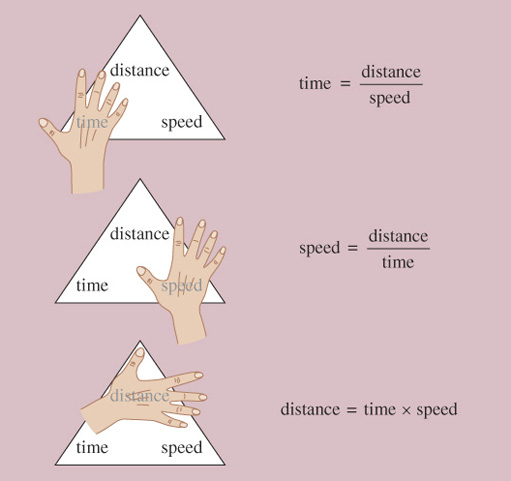Figure 28 Using the formula triangle

## Activity 29

In Activity 20 you drew a formula triangle for the relationship between battery capacity, current (i) and the length of time a battery can be used (t).

1. Suppose you know the battery capacity and the time you want to use the battery for. Write down the formula which will allow you to calculate the current. Use the formula triangle to help you.

2. Suppose a battery has a capacity of 1.8 Ah and is to be used for 20 hours. What is the maximum current that can be drawn from it?

### Discussion

1. You need to use the formula triangle from the answer to Activity 20. If you know time and capacity, you can see by covering i (current) that current will be given by: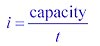2. If the capacity is 1.8 Ah and the time is 20 hours, the maximum current is: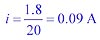It is important when doing the calculations that you use consistent units. You could use the standard units known as SI units (see box below), but you don't have to, so long as the units are consistent. For example, if you have speed in kilometres per hour and time in hours, then distance will be in kilometres.

SI units

One way of ensuring that you are working with consistent units is to use the international standard units known as 'SI' units, where SI stands for the French words Système International. The SI unit for length is the metre and for time is the second. In SI units, therefore, speed is expressed as metres per second. There is more about SI units in The Sciences Good Study Guide (Northedge et al., 1997).

## Activity 30

For a communications satellite to be in a geostationary orbit it has to be about 36,000 km above the Earth. How much delay will be introduced to a radio signal by having to go up to and back down from the satellite? Radio signals travel at the speed of light (3×108 m/s), and you should assume that the signals go straight up and straight down. Note that this assumption – straight up and straight down – simplifies the calculation, and means that you get a value that would be an underestimate to the delay, for all cases except where the communication really is straight up and down (see Figure 29). In practice, communication via the satellite will often use an angled path and therefore have a larger delay.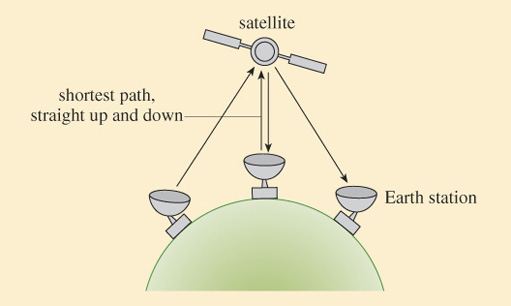Figure 29 Path lengths in satellite communications

### Discussion

We need the time, so we use: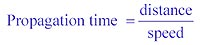We first write the distance and speed in consistent units. The speed is in units of metres per second (3×108 m/s) but the distance is in kilometres (36,000 km). The multiplier 'kilo' is ×1000, so in metres the distance is 36,000×1000 m=36,000,000 m=3.6×107 m. This is the distance to or from the satellite. One 'hop' – up and down – is twice this distance, 7.2×107m.

So we have: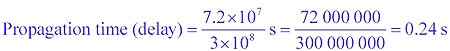You might think that 0.24 seconds (about a quarter of a second) is not very long, but in fact if you were having a conversation with someone and there was a delay this long, it would be quite noticeable – and something of a nuisance. When you say something then stop to wait for a reply, it takes a quarter of a second for what you say to reach the other person, then there is another quarter of a second delay before the reply reaches you. So, in total, there is half a second delay (in addition to the recipient deciding what to say) between you finishing what you say and hearing the reply.

## Activity 31

Satellites are frequently used for transatlantic communication, but the alternative is to use undersea cables. These days undersea cables would invariably use optical fibre. Light in fibre travels at about 2/3 of the speed of light in the air (light travels more slowly in glass than in the air) and therefore the signal speed is about 2×108 m/s. The distance across the Atlantic (depending upon where you start and finish) is about 4000 km. How long does it take for a signal to cross the Atlantic travelling through optical fibre?

### Discussion

We need the time, so we use: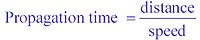We first write the distance and speed in consistent units. The speed is in metres per second, m/s. The distance is in kilometres, so we need to change it to metres. 1 kilometre is 1000 metres, so 4000 km is 4000 × 103 m=4×106 m.

So,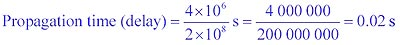You will have seen from the last activity that the delay when using optical fibre is very much less than when using a geostationary satellite. This is not the whole story, because there may be further delays when signals are manipulated (which can happen both with satellites and optical fibre links), but nevertheless it remains true that in speech telephony there is a noticeable delay when the communication uses a geostationary satellite, but not, usually, when it uses a fibre link. Delays used to be commonly encountered when you telephoned the USA from the UK, but that is rare these days because most transatlantic calls are via optical fibre links. It is important to appreciate that the large delay when using geostationary satellites comes about because geostationary satellites are so far from the Earth. Other satellites are also used whose orbits are much closer to the Earth. Communication via these non-geostationary satellites can have a smaller delay, but there are other complications because the satellite is moving relative to the Earth's surface.

As you saw in the Taylor extract in Section 2, news broadcasts often use geostationary satellites and therefore suffer from the larger delay. The processing (manipulation) used in MPEG encoding, especially motion compensation, adds yet more delay. The combined delays of transmission and processing can cause problems for live news broadcasts, as Higgins discusses later in his book:

The interaction in a 'live' two-way interview requires that both the questions and answers are delivered as smoothly as possible, but the delay of the compression process which is then added to the fixed satellite delay means that these interviews often have an awkward hesitancy about them.

This can be masked to a degree by imaginative techniques used both in the studio and out in the field, but there is always the evident hesitation between interviewer and interviewee. These compression delays ('latency') have reduced as the computational advances in processing speed have increased.

The viewer is also growing more tolerant of these delays to the point where they are hardly noticed, and so the problems are diminishing as time passes.

Some coders also offer a facility to improve the latency at the expense of movement compensation [motion compensation] – the so-called interview or low-delay mode. This is selected via the front panel menu of the MPEG-2 coder, reducing the overall processing time of the signal.

Changing production techniques is by far the best way to try to overcome these awkward pauses. It is common for the studio to cue the reporter a fraction of a second earlier than normal, so that by the time they respond, the delay has passed unnoticed.

Often you will see reporters in the field looking thoughtful or slowly nodding after they have replied to a question, so that it makes their eventual answer to the next question look as if it is very carefully considered reply! Like many other things in TV, much can be achieved by using 'smoke and mirrors'!

These techniques of course do not work if it is a straight 'down the line' interview with a member of the public, who naturally is unaware of these tricks of the trade.

However, you can often see this all going horribly wrong even with a seasoned reporter if there is a studio presenter asking questions who does not appreciate the subtle techniques required to cope with satellite and compression delay.

Classically this happens when part way through the answer from a reporter in the field, the presenter interjects with a supplementary question or comment. The field reporter carries on for a second or so, then halts in their tracks, meanwhile the studio presenter realises their mistake and urges the reporter in the field to continue – and you get a cycle of each end saying 'Sorry, please go on'.

This was a lesson that had to be learnt in the early days of satellite broadcasting which became more acute when digital processing and encoding was introduced – yet you still see these problems occurring today.

Higgins (2004)
T175_8